×#### Thank you for registering.

One of our academic counsellors will contact you within 1 working day.

Click to Chat

1800-1023-196

+91-120-4616500

CART 0

• 0

MY CART (5)

Use Coupon: CART20 and get 20% off on all online Study Material

ITEM
DETAILS
MRP
DISCOUNT
FINAL PRICE
Total Price: Rs.

There are no items in this cart.
Continue Shopping• Complete JEE Main/Advanced Course and Test Series
• OFFERED PRICE: Rs. 15,900
• View Details

```Chapter 7: Trigonometric Ratios of Compound Angles – Exercise 7.2

Trigonometric Ratios of Compound Angles – Exercise 7.2 – Q.1

Let f(θ) = 12 sin θ - 5 cos θ

We know that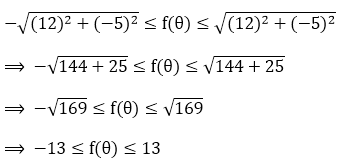Hence, minimum and maximum values of 12 sin θ - 5 cos θ are -13 respectively.

Let f(θ) = 12 cos θ + 5 sin θ + 4

We know that⟹ -13 ≤ 12 cos θ + 5sin θ ≤ 13

⟹ -13 + 4 ≤ 12 cos θ + 5sinθ + 4 ≤ 13 + 4

⟹ -9 ≤ 12 cos θ + 5sinθ + 4 ≤ 17

⟹ -9 ≤ f(θ) ≤ 17

Hence, minimum and maximum values of 12 cos θ + 5 sin θ + 4 are – 9 and 17 respectively.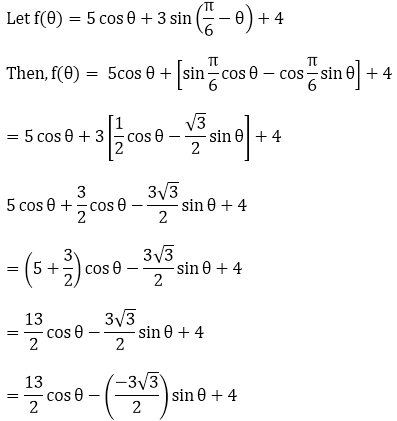We know that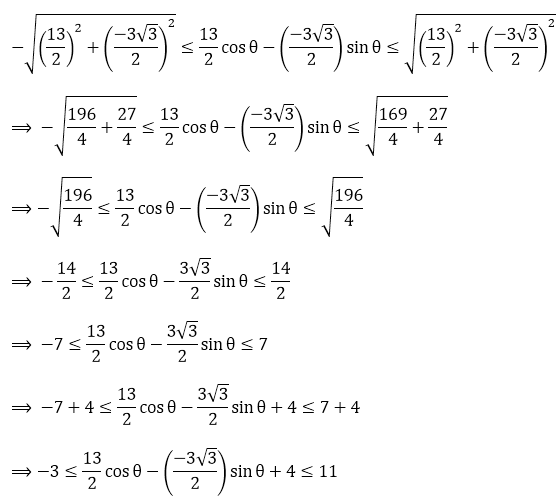⟹ -3 ≤f (θ) ≤ 11

Let f(θ) = sin θ - cos θ + 1. Then,

f(θ) = sin θ + (-1) cos θ + 1

= (-1) cos θ + sin θ + 1

We know that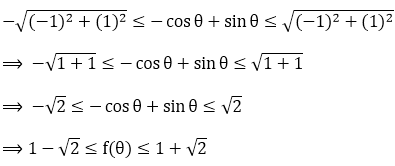Hence, minimum and maximum values of sin θ - cos θ + 1 are 1 - √2  and 1 + √2 respectively.

Trigonometric Ratios of Compound Angles – Exercise 7.2 – Q.2

Let f(θ) = √3 sin θ - cos θ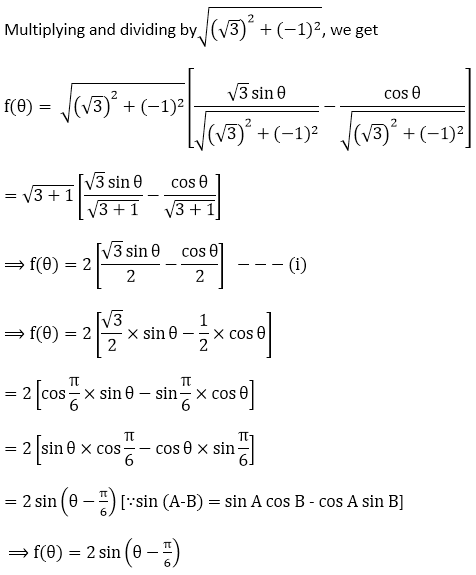Again,Let f(θ) = cos θ - sin θAgain,Let f(θ) = 24 cos θ + 7 sin θAgain,Trigonometric Ratios of Compound Angles – Exercise 7.2 – Q.3

We have

sin100° - sin10°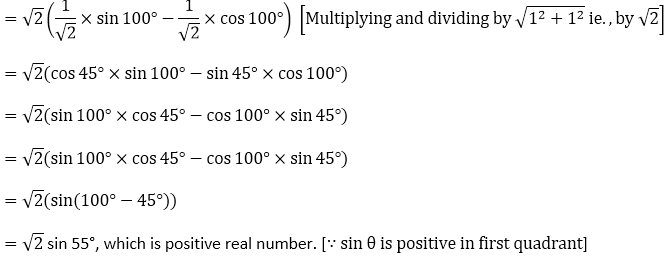Trigonometric Ratios of Compound Angles – Exercise 7.2 – Q.4Dividing and multiplying the above equation with above valueWe know that maximum and minimum value of any cosine term is +1 and -1```### Course Features

• 728 Video Lectures
• Revision Notes
• Previous Year Papers
• Mind Map
• Study Planner
• NCERT Solutions
• Discussion Forum
• Test paper with Video Solution